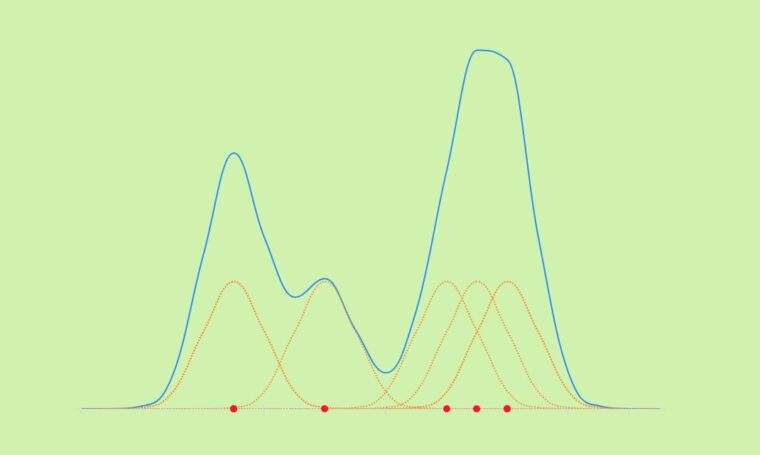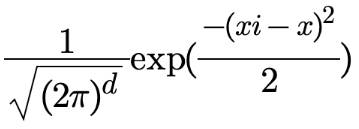# How to create an interactive kernel density chart

2020-11-06 22:21:56 roffey

Highcharts It's a pure JavaScript A library of charts compiled , For you Web Website 、Web Applications provide intuitive 、 Interactive charts . Currently, broken lines are supported 、 curve 、 Area 、 Regional plot 、 Bar charts 、 Bar chart 、 The pie chart 、 Scatter plot 、 Angle measurement chart 、 Area map 、 Regional curve arrangement 、 Column arrangement 、 There are dozens of chart types, such as polar charts .Kernel density estimation is a useful statistical method , Used to estimate the overall shape of the distribution of random variables . let me put it another way , Nuclear density estimates （ Also known as KDE） Can help us “ smooth ” And exploration does not follow any typical probability density distribution （ For example, normal distribution , Binomial distribution, etc ） The data of .

In this tutorial , We're going to show you how to Javascript Create interactive kernel density estimation and use Highcharts Library drawing results .

Let's first explore KDE chart ; Then we're going to delve into the code .

The following demonstration shows the Gaussian kernel density estimation for random data sets ：This chart helps us estimate the probability distribution of random data sets , And we can see that the data is mainly concentrated at the beginning and end of the chart .

Basically , For each red data point , We draw a Gaussian kernel function in orange , Then sum all the kernel functions together , Create density estimates in blue （ Please see the demo）：By the way , There are many kernel function types , For example, Gauss , Unified ,Epanechnikov etc. . We're using a Gaussian kernel , Because it provides a smooth pattern .
The mathematical expression of Gaussian kernel is ：Now? , You have an idea of what the kernel density estimation looks like , Let's take a look at the code behind it .
There are four main steps in the code ：

1. Create a Gaussian kernel function .
2. Processing density estimators .
3. Dealing with kernel points .
4. Plot the entire data point .

Gaussian kernel

The following code represents the Gaussian kernel function ：

```function GaussKDE(xi, x) {
return (1 / Math.sqrt(2 * Math.PI)) * Math.exp(Math.pow(xi - x, 2) / -2);
}```

among x Represents the main data （ Observed value ）,xi Represents the range and density estimation function of the rendering kernel . In our case ,xi The scope is 88 To 107, To ensure coverage 93 To 102 The range of observational data for .

Density estimation point

Here's how to recycle GaussKDE() Array representation of functions and ranges to create density estimators

```xiData：
//Create the density estimate
for (i = 0; i < xiData.length; i++) {
let temp = 0;
kernel.push([]);
kernel[i].push(new Array(dataSource.length));
for (j = 0; j < dataSource.length; j++) {
temp = temp + GaussKDE(xiData[i], dataSource[j]);
kernel[i][j] = GaussKDE(xiData[i], dataSource[j]);
}
data.push([xiData[i], (1 / N) * temp]);
}```

Kernel point

Only if you want to show kernel points （ Orange chart ） You need to perform this step when . otherwise , You are already satisfied with the density estimation steps . This is the code that handles the data points of each kernel ：

```//Create the kernels
for (i = 0; i < dataSource.length; i++) {
kernelChart.push([]);
kernelChart[i].push(new Array(kernel.length));
for (j = 0; j < kernel.length; j++) {
kernelChart[i].push([xiData[j], (1 / N) * kernel[j][i]]);
}
}```

Basically , This loop just adds the scope xiData To kernel Each array that has been processed in the density estimation step .

Draw points

After processing all the data points , You can use Highcharts Rendering series . Density estimates and kernels are spline type , The observations are plotted as scatter plots ：

```Highcharts.chart("container", {
chart: {
type: "spline",
animation: true
},
title: {
text: "Gaussian Kernel Density Estimation (KDE)"
},
yAxis: {
title: { text: null }
},
tooltip: {
valueDecimals: 3
},
plotOptions: {
series: {
marker: {
enabled: false
},
dashStyle: "shortdot",
color: "#ff8d1e",
pointStart: xiData,
animation: {
duration: animationTime
}
}
},
series: [
{
type: "scatter",
name: "Observation",
marker: {
enabled: true,
fillColor: "#ff1e1f"
},
data: dataPoint,
tooltip: {
pointFormat: "<b>{point.x}</b>"
},
zIndex: 9
},
{
name: "KDE",
dashStyle: "solid",
lineWidth: 2,
color: "#1E90FF",
data: data
},
{
name: "k(" + dataSource + ")",
data: kernelChart
},...  ]
});```

Now? , You are going to explore your own data using the function of the kernel density estimation map .